GrindEQ Math UtilitiesConverting solutions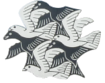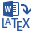Word-to-LaTeX converts Microsoft Word documents to LaTeX, AMS-LaTeX, Plain TeX, or AMS-TeX format.

Convert your Microsoft Word documents to LaTeX or TeX;

Convert equations (Microsoft Equation, Equation Editor 3.x, and MathType) in editable form;

Prepare equations for publication on the Internet using the optional MathJax compatibility mode;

Convert tables, lists, headers/footers, cross-references, etc.;

Export images in PS/EPS/PNG/JPEG format;

ANSI, OEM, MAC, Unicode, and UTF-8 encoding are supported;

Convert a whole Microsoft Word document or a selected part.

See more...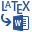LaTeX-to-Word converts LaTeX, AMS-LaTeX, Plain TeX, or AMS-TeX documents to Microsoft Word format.

Convert your TeX and LaTeX documents to Microsoft Word;

Choose either Microsoft Equation, Equation Editor 3.x, or MathType format for converted equations;

BibTeX emulator is included;

LaTeX cross-referencing and Microsoft Word cross-referencing fields are supported;

Import images, including PostScript;

ANSI, OEM, MAC, Unicode, and UTF-8 encoding are supported;

Convert a whole LaTeX document or a selected part.

See more...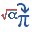MathType-to-Equation converts MathType and Equation Editor 3.x objects to Microsoft Equation format.

Edit MathType equations in Microsoft Equation Editor;

Update your old equations to new format (Equation3-to-Equation is included);

Enable/disable Euclid fonts;

Convert a whole Microsoft Word document or selected equations.

See more...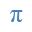Equation3-to-Equation converts old Equation Editor 3.x objects to Microsoft Equation format.

Update your old equations to new format;

Equation3-to-Equation is a part of MathType-to-Equation.

See more...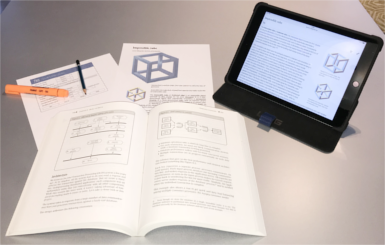Image-to-PostScript

This freeware utility extracts Microsoft Word graphical objects (images, pictures, raster/vector graphics, diagrams, etc.) and creates PostScript files, which can be inserted into TeX/LaTeX document.

See more...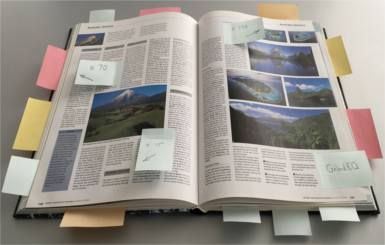Cross-references

Cross-referencing is an essential aspect of professionally prepared documents. References can be maintained manually (as most of the Microsoft Word users are used to do), but with the document size growth the procedure of maintaining references becomes a quite time-consuming task. And it would be a nice idea to automate such a tedious routine. The freeware GrindEQ Cross-references utilities provide incredibly easy tools to do this.

See more...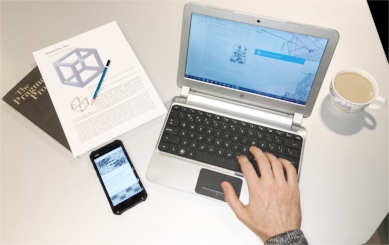Other tools

With GrindEQ Math Utilities you can change Equation Editor 3.x appearance to MathType style: Equation Editor 3.x will start in its own window, so you will be able to select different view zoom and edit several equations simultaneously. The Normalizer utility normalizes Equation Editor 3.x objects to the same appearance (e.g. the same font, style, and size).